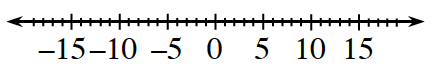### Home > CC2MN > Chapter 6 Unit 6B > Lesson CC2: 6.1.1 > Problem6-11

6-11.

Copy each part below on your paper. Then use the number line to help you fill in $<$ (less than) or $>$ (greater than) on the blank line. Homework Help ✎Numbers increase from left to right and decrease from right to left.

1. $-5$            $-2$

Locate $−5$ and $−2$ on the number line.

Since $−5$ is to the left of $−2$ and numbers decrease from right to left, that means to $−5$ is less than $−2$.

1. $8$            $-1$

See part (a).

1. $-5$            $0$

See part (a).

1. $-15$            $-14$

See part (a).